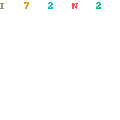# Bifurcation and Chaos in Discontinuous and Continuous SystemsBifurcation and Chaos in Discontinuous and Continuous Systems by Michal Fečkan
English | PDF | 2011 | 387 Pages | ISBN : 3642182682 | 1.41 MB

“Bifurcation and Chaos in Discontinuous and Continuous Systems” provides rigorous mathematical functional-analytical tools for handling chaotic bifurcations along with precise and complete proofs together with concrete applications presented by many stimulating and illustrating examples. A broad variety of nonlinear problems are studied involving difference equations, ordinary and partial differential equations, differential equations with impulses, piecewise smooth differential equations, differential and difference inclusions, and differential equations on infinite lattices as well.

This book is intended for mathematicians, physicists, theoretically inclined engineers and postgraduate students either studying oscillations of nonlinear mechanical systems or investigating vibrations of strings and beams, and electrical circuits by applying the modern theory of bifurcation methods in dynamical systems.

Dr. Michal Fečkan is a Professor at the Department of Mathematical Analysis and Numerical Mathematics on the Faculty of Mathematics, Physics and Informatics at the Comenius University in Bratislava, Slovakia. He is working on nonlinear functional analysis, bifurcation theory and dynamical systems with applications to mechanics and vibrations.